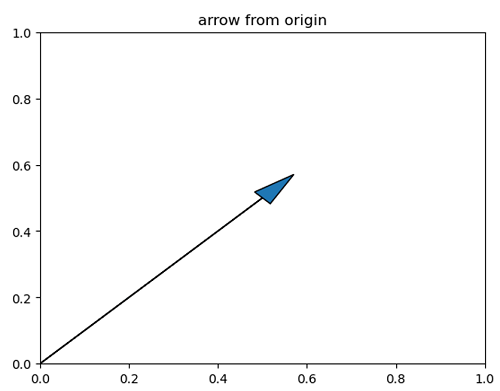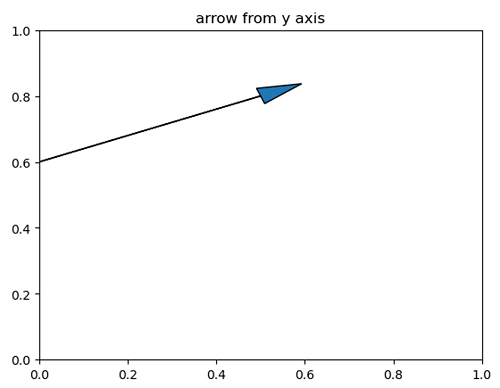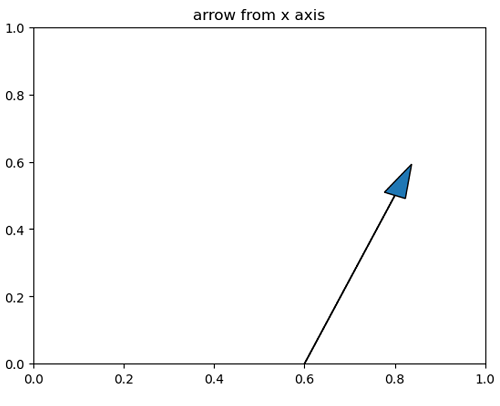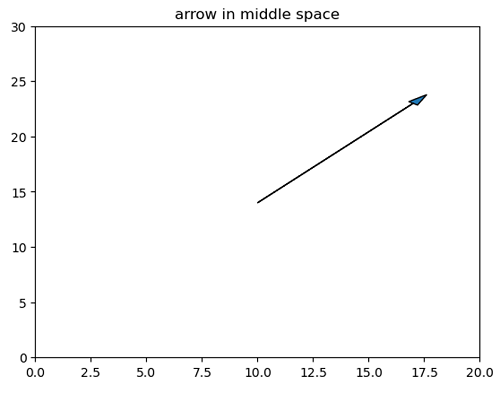# Python | Arrow in Plotting

Arrow in Plotting in Python. Here, we are going to learn about the arrow in plotting and its Python implementation.
Submitted by Anuj Singh, on July 20, 2020

In this tutorial, we are going to learn how to add an arrow to a plot? Matplotlib has provided an inbuilt function for plotting an arrow matplotlib.pyplot.arrow().

The following example illustrates the implementation and use of the Arrow.

```# Arrow starting from origin
# Arrow tip point :(x,y) = (0.5,0.5)
```## Python code for arrow in plotting

```import matplotlib.pyplot as plt

plt.figure()
plt.title('arrow from origin')
plt.show()

plt.figure()
plt.title('arrow from y axis')
plt.show()

plt.figure()
plt.title('arrow from x axis')
plt.show()

plt.figure()
plt.xlim(0,20)
plt.ylim(0,30)
plt.title('arrow in middle space')
plt.show()
```

Output:

```Output is as figure
```

Languages: » C » C++ » C++ STL » Java » Data Structure » C#.Net » Android » Kotlin » SQL
Web Technologies: » PHP » Python » JavaScript » CSS » Ajax » Node.js » Web programming/HTML
Solved programs: » C » C++ » DS » Java » C#
Aptitude que. & ans.: » C » C++ » Java » DBMS
Interview que. & ans.: » C » Embedded C » Java » SEO » HR
CS Subjects: » CS Basics » O.S. » Networks » DBMS » Embedded Systems » Cloud Computing
» Machine learning » CS Organizations » Linux » DOS
More: » Articles » Puzzles » News/Updates